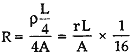Monday , May 23 2022# Electricity: 10th CBSE Science Physics

#### Question: There are two electric bulbs, (i) marked 60 W, 220 V and (ii) marked 100 W, 220 V. Which one of them has higher resistance?

Answer: We know that R = V²/p or R ∞ 1/p. Thus, 60 W lamp has more resistance than a 100 W lamp.

#### Question: Out of the two, a toaster of 1 kW and an electric heater of kW, which has greater resistance?

Answer: We know that R = V²/p or R ∞ 1/p. Thus, 1 kW toaster, has more resistance than a 2 kW electric heater.

#### Question: Why are alloys commonly used in electrical heating devices?

Answer: This is because their resistivity is high and they do not oxide readily at high temperatures.

#### Question: What is the resistance of an electric arc lamp, if the lamp uses 20 A when connected to 220 volt line?

Answer: R = V/I = 220/20 =11 ohm

#### Question: Read the following statements carefully and write whether the statement is true or false:

1. The quantity of charge flowing past a point multiplied by time is current.
2. The flow of charge through a conducting wire connected to a cell is the result of chemical reaction inside the cell.
3. The resistivity of all pure metals increases with increase in temperature.
4. Ohm’s law is a relation between the power used in a circuit to the current and the potential difference.
5. A series circuit has only one conducting path for the electrons that move through it; a parallel circuit has multiple conducting paths.
6. A conducting wire offers resistance to the flow of electrons because electrons repel each other in the wire.

1. False
2. True
3. True
4. False
5. True
6. False

#### Question: Does an ammeter have a low or a high resistance?

Answer: An ammeter has a low resistance.

#### Question: Why is an ammeter likely to be burnt, if you connect it in parallel?

Answer: If an ammeter is connected in parallel, the resultant resistance of the circuit decreases and more current passes through the instrument. Hence, the ammeter is likely to be burnt out.

#### Question: What is the resistance of an ideal ammeter?

Answer: An ideal ammeter has zero resistance.

#### Question: What is the resistance of an ideal voltmeter?

Answer: An ideal voltmeter has infinite resistance.

#### Question: What can be the different between the specific resistance for a thin wire made of the same material?

Answer: The specific resistance of a thin and thick wire will be same.

#### Question: A bulb gets dimmer for a moment when a geyser, connected across the same source is switched on. Why?

Answer: The geyser draws a heavy current for a moment which caused potential drop in the line. Due to this, bulb gets dimmer.

#### Question: Why is very less heat generated in long electric cables than in filaments of electric bulbs?

Answer: It is because resistance of filament of electric bulb is much more than that of electric cable.

#### Question: Define electric circuit. Distinguish between open and closed circuit.

Answer: It is an arrangement in which an electric current can flow. When the switch is on, the circuit is closed and when the switch is off, the circuit is open.

#### Question: What is the lowest resistance that can be obtained by combining four coils of resistances 4 Ω, 8 Ω, 12 Ω and 24 Ω ?

Answer: By combining them in parallel. The lowest resistance which can be obtained is 2 Ω.

#### Question: Nichrome is used to make the element of electric heater. Why?

Answer: It is an alloy with high resistivity and high melting point.

#### Question: What happens to the resistance of a conductor when the length of the conductor is reduced to half?

Answer: When length is reduced to half, resistance also reduces to half of its original value.

#### Question: Why do we use copper and aluminium wire for transmission of electric of electric current?

Answer: These have low resistivity and are good conductor of electricity.

#### Question: A given length of a wire is doubled on itself and this process is repeated once again. By what factor does the resistance of the wire change?

Answer: Length L/4 and area 4 timesHence, the resistance changes by 1/16 times.

## Sources of Energy: 10th Science Chapter 14

Class: 10th Class Subject: Science Chapter: Chapter 14: Sources of Energy Quiz: – Questions MCQs: …